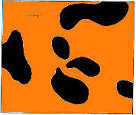# The Tiger's Stripes

A technical blog on math, computer science, and game theory.

Author: Bo Waggoner RSS feed# Divergences and Value of Information

Posted: 2016-10-07.

This is a follow-up to generalized entropies and the value of information, where we discussed how value of information connects to generalized entropies. Here, we'll connect both to Bregman divergences, filling in a third side of the triangle.

The goal is to complete a set of three equivalent but intuitively distinct answers to the question:

How do we measure the amount of information conveyed by a signal (random variable) $A$?

Our answer, for a given decisionmaker, is three equivalent ways to measure amount or value of information.

Informal equivalence. A decisionmaker can be captured by a value-of-information function, a divergence, and an entropy function such that:

Very valuable information (according to your utility function) moves your beliefs a large distance (according to your divergence measure) and also reduces a lot of uncertainty (according to your entropy measure).

Imagine for a moment you're a perfect Bayesian reasoner. ("Imagine? what does he mean imagine?")

You have a belief $p$ about some fact or event, e.g. whether My Favorite Sports Team wins the Big-O Derby Cup this year. Then you get a piece of new information -- maybe their star player injured her lateralus. How much does your belief change?

We can measure such a change with a distance function $D(q,p)$ from your posterior belief $q$ to your prior belief $p$. For example, you might use KL-divergence $KL(q,p) = \sum_x q(x) \log \frac{q(x)}{p(x)}$.

A nice generalization of KL-divergence is the Bregman divergence corresponding to a convex function $G$, namely $D_G(q,p) := G(q) - LA(p,q)$ where $LA(p,q)$ is not a city but a linear approximation: $LA(p,q) = G(p) + \langle G'(p), q-p \rangle .$ The KL-divergence is a special case corresponding to the function $-H(p) = \sum_x p(x) \log p(x)$.

(There are two reasons I'm ordering the arguments as $D_G(q,p)$ rather than $D_G(p,q)$. First, it seems that $D_G(a,b)$ measures in a sense the difference between a "correct" $a$ and an "incorrect" approximation $b$. So it makes more sense to stand at the posterior belief $q$, look back at the prior, and ask how "wrong" it was or how far it "diverged". Second, it meshes with the story I'm about to tell.)

## Recap

Recall from generalized entropies and the value of information that a decision problem consists of a utility function $u$ and a random variable $E$. The decisionmaker picks a decision $d$ and gets expected utility $\mathbb{E} u(d, e)$ where $e$ is an outcome of $E$. For instance, $E$ could be whether or not MFS Team wins the Cup this year, and the decision could be what bet to place on them.

The first thing we said was that there exists a convex function $G$ such that, for any distribution $p$ on $E$, the expected utility for acting optimally in the face of that distribution is $G(p)$. We can overload notation by writing $G(E)$ for this same quantity.

Then we supposed that the decisionmaker first observes a signal $A$ as a random variable that is correlated with $E$ (such as learning whether or not the star player is injured). We wrote $G(E|A)$ for the expected utility for deciding given access to $A$. So the marginal value of $A$ was measured by $G(E|A) - G(A)$, where $G(E|A) = \mathbb{E}_a G(p_a)$.

Meanwhile, we said that one can use a (generalized) entropy function $F$ to measure the information that $A$ conveys about $E$, which was $F(E) - F(E|A)$. Since I defined generalized entropy functions to simply be concave functions, we have a correspondence between entropies and decision problems via $F = -G$.

## Measuring Value By Change

Ok, here's the point.

Fact. Consider any Bregman divergence $D_G$ for convex $G$. Let $p$ be the prior on $E$, $A$ a signal, and $p_a$ the posterior on $E$ given $A=a$.
Then the expected change in posterior belief $\mathbb{E}_{a} D_G(p_a, p)$ is equal to the marginal value $G(E|A) - G(E)$, also equal to the reduction in entropy $F(E) - F(E|A)$ for generalized entropy $F = -G$.

Proof. Exercice!

To me, this is interesting/important because it paints the same picture as the previous blog post -- amount of information revealed by a signal -- in a very different light. Hopefully it can lead to actually interesting and useful applications as well, but we'll have to see!

Limits to 1000 characters. HTML does not work but LaTeX does, e.g. $\$$x^y\$$ displays as$x^y\$.